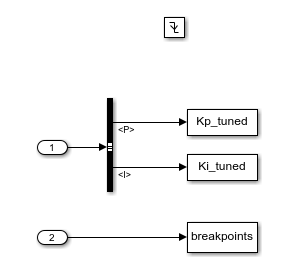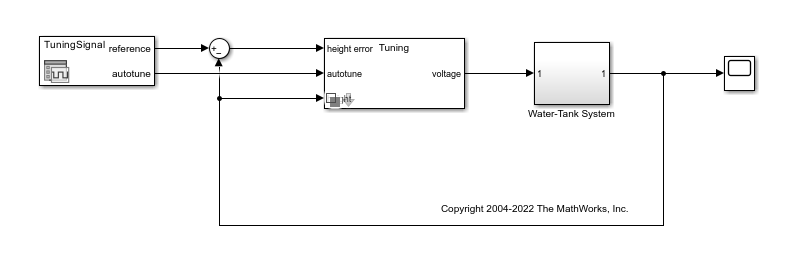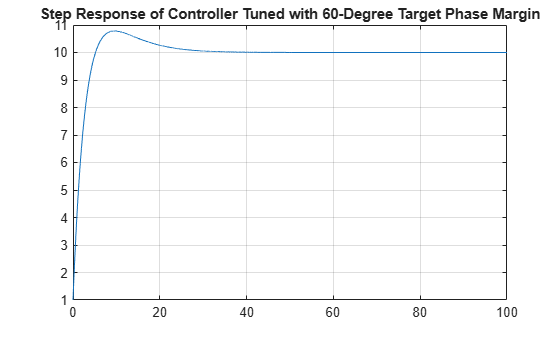# Tune Gain-Scheduled Controller Using Closed-Loop PID Autotuner Block

This example shows how to use the Closed-Loop PID Autotuner block to tune a gain-scheduled controller in one simulation.

### Water-Tank System Model

This example uses a gain-scheduled controller to control the water level of a nonlinear Water-Tank System plant. The Water-Tank System plant is originally controlled by a single PI controller in the `watertank` Simulink® model. For more details on the nonlinear Water-Tank System plant, see watertank Simulink Model.The following sections describe how to modify the `watertank` model for tuning and validating gain-scheduled controller. Alternatively, use the `watertank_gainscheduledcontrol` model provided with this example.

#### Connect Closed-Loop PID Autotuner Block with Plant and Controller

Insert the Closed-Loop PID Autotuner block between the controller and plant as shown in the following diagram. The start/stop signal starts and stops the closed-loop experiment. When no experiment is running, the Closed-Loop PID Autotuner block behaves like a unity gain block, where the u signal passes directly to u+Δu.#### Connect Blocks to Store Tuned Gains

To create a gain schedule, the autotuned gains are recorded at each operating point. In this example, a triggered subsystem is used to write the reference heights and controller gains to the workspace upon falling edges of the autotuner start/stop signal. Simulating this model produces an array of tuned gains and breakpoints for easy use with dynamic lookup tables to test the controller.#### Validate Performance of Gain-Scheduled Controller

After you obtain a set of breakpoints and tuned gains, test the tuned gain-scheduled controller with the Water-Tank System plant. To do so, remove the autotuner block, change the source of the PID Controller block to external, and insert Lookup Table Dynamic blocks as shown in the diagram.#### Integrate Both Tuning and Testing in Example Model

In this example, a gain-scheduled controller is tuned using the Closed-Loop PID Autotuner block and its performance is then tested in the same model. The example model uses a variant subsystem to organize the tuning and testing workflows.To switch between Tuning and Testing modes, double-click the Variant Subsystem block.### Tune Controller at Single Operating Point

Before tuning the gain-scheduled controller at multiple operating points, tuning at single operating point helps you configure the Closed-Loop PID Autotuner block. Open the example model `watertank_gainscheduledcontrol` with controller gains used by the `watertank` Simulink model.

```mdl = 'watertank_gainscheduledcontrol'; Kp = 1.599340; Ki = 0.079967; open_system(mdl); set_param([mdl,'/Variant Subsystem'],'SimMode','Tuning');```

#### Configure Closed-Loop PID Autotuner Block

After connecting the Closed-Loop PID Autotuner block with the Water-Tank System plant model and PID Controller block, use the block parameters to specify tuning and experiment settings. This example uses the same design requirements found in the example Design Compensator Using Automated PID Tuning and Graphical Bode Design. These design requirements are in the form of closed-loop step response characteristics.

• Overshoot less than 5%

• Rise time less than 5 seconds

To tune the PID controller to meet the above design requirements, parameters of the Closed-Loop PID Autotuner block are pre-populated. The Tuning tab has three main tuning settings.

• Target bandwidth — Determines how fast you want the controller to respond. The target bandwidth is roughly 2/desired rise time. For a desired rise time of 4 seconds, set target bandwidth = 2/4 = 0.5 rad/s.

• Target phase margin — Determines how robust you want the controller to be. In this example, start with the default value of 60 degrees.

• Experiment sample time — Sample time for the experiment performed by the autotuner block. Use the recommended 0.02/bandwidth for sample time = 0.02/0.5 = 0.04s.

The Experiment tab has three main experiment settings.

• Plant Type — Specifies whether the plant is asymptotically stable or integrating. In this example, the Water-Tank System plant is integrating.

• Plant Sign — Specifies whether the plant has a positive or negative sign. The plant sign is positive if a positive change in the plant input at the nominal operating point results in a positive change in the plant output when the plant reaches a new steady state. In this example, the Water-Tank System plant has a positive plant sign.

• Sine Amplitudes — Specifies amplitudes of the injected sine wave perturbations. In this example, specify a sine amplitude of 0.3.

#### Simulate at One Operating Point

Start the experiment at 140 seconds to ensure that the water level has reached steady-state $H=10$. The recommended experiment duration is 200/bandwidth seconds = 200/0.4 = 500s. With start time of 140 seconds, the stop time is 640 seconds. The simulation stop time is further increased to capture the full experiment.

```set_param([mdl,'/Variant Subsystem/Tuning/Closed-Loop PID Autotuner1'],'TargetPM','60'); set_param([mdl,'/Signal Editor'],'ActiveScenario','TuningSignal_OnePoint'); simOut = sim(mdl,'StopTime','800'); simOut.Kp_tuned```
```ans = 1.8254 ```
`simOut.Ki_tuned`
```ans = 0.2037 ```

In the `watertank` Simulink model, initial PI controller gains are Kp = 1.599340 and Ki = 0.079967. After tuning, the controller gains are Kp = 1.82567 and Ki = 0.20373.

#### Check Tuning Result and Adjust Autotuning Parameters

Replace controller gains with the new autotuned gains and validate the design requirements.

```Kp = simOut.Kp_tuned; Ki = simOut.Ki_tuned; simOut = sim(mdl,'StopTime','100'); figure; plot(simOut.ScopeDataGS.time,simOut.ScopeDataGS.signals.values); grid on title('Step Response of Controller Tuned with 60-Degree Target Phase Margin');``````StepPerformance_OnePoint = stepinfo(simOut.ScopeDataGS.signals.values(:), ... simOut.ScopeDataGS.time(:),10,1)```
```StepPerformance_OnePoint = struct with fields: RiseTime: 3.6254 TransientTime: 22.5227 SettlingTime: 22.5227 SettlingMin: 9.1086 SettlingMax: 10.7822 Overshoot: 8.6912 Undershoot: 0 Peak: 9.7822 PeakTime: 9.5500 ```

The step response has a rise time of 3.6251 seconds and overshoot of 8.6895%. The overshoot is larger than desired; increase target phase margin to 75 degrees to improve the closed-loop transient response.

`set_param([mdl,'/Variant Subsystem/Tuning/Closed-Loop PID Autotuner1'],'TargetPM','75');`

Examine the simulation result. The system is at steady-state when experiment starts and returns to steady-state after tuning is completed. As an indication of controller tuning performance, the Closed-Loop PID Autotuner block reaches 100% convergence level sooner than the recommended 500 seconds. As a result, reduce experiment duration to 300 seconds, meaning a stop time of 440 seconds. Accordingly, decrease the simulation stop time from 800 seconds to 500 seconds.

```Kp = 1.599340; Ki = 0.079967; set_param([mdl,'/Signal Editor'],'ActiveScenario','TuningSignal_OnePointAdjusted'); simOut = sim(mdl,'StopTime','500'); simOut.Kp_tuned```
```ans = 1.9348 ```
`simOut.Ki_tuned`
```ans = 0.1142 ```

Simulating with new experiment parameters produces tuned gains of Kp = 1.93514 and Ki = 0.11415. Examine the step response again using gains tuned with the increased target phase margin value.

```Kp = simOut.Kp_tuned; Ki = simOut.Ki_tuned; simOut = sim(mdl,'StopTime','100'); figure; plot(simOut.ScopeDataGS.time,simOut.ScopeDataGS.signals.values); grid on title('Step Response of Controller Tuned with 75-Degree Target Phase Margin');``````StepPerformance_OnePointAdjusted = stepinfo(simOut.ScopeDataGS.signals.values(:), ... simOut.ScopeDataGS.time(:),10,1)```
```StepPerformance_OnePointAdjusted = struct with fields: RiseTime: 4.1402 TransientTime: 21.4152 SettlingTime: 21.4152 SettlingMin: 9.1041 SettlingMax: 10.2832 Overshoot: 3.1463 Undershoot: 0 Peak: 9.2832 PeakTime: 12.1100 ```

The step response has a rise time of 4.1398 seconds and overshoot of 3.1438%, both of which meet the design requirements.

Simulate the model with tuned gains for multiple operating points $H$ = [5, 10, 15, 20].

```set_param([mdl,'/Signal Editor'],'ActiveScenario','TuningSignal_SinglePID'); simOut_single = sim(mdl,'StopTime','2400');```

### Tune Gain-Scheduled Controller at Multiple Operating Points

The set of tuned gains produces a desired response. You can now perform tuning at multiple operating points to create a gain-scheduled controller.

#### Create Input Tuning Signal

The operating range of scheduling variable $H$ from 1 to 20 is covered by the operating points for autotuning. In this example, the gain-scheduled controller gains are tuned at four operating points with $H$ = [5, 10, 15, 20]. To tune at multiple operating points, use the Signal Editor block to create the reference and autotuner start/stop signal#### Simulate Multiple Operating Points

Using the input signal, simulate the `watertank_gainscheduledcontrol` model for the entire length of the autotuning process. At the end of simulation, save both tuned gains and breakpoints as vectors in the MATLAB® Workspace.

```Kp = 1.599340; Ki = 0.079967; set_param([mdl,'/Signal Editor'],'ActiveScenario','TuningSignal'); simOut = sim(mdl,'StopTime','2400'); Kp_tuned = simOut.Kp_tuned```
```Kp_tuned = 4×1 1.9279 1.9327 1.9358 1.9380 ```
`Ki_tuned = simOut.Ki_tuned`
```Ki_tuned = 4×1 0.1277 0.1183 0.1122 0.1078 ```
`breakpoints = simOut.breakpoints`
```breakpoints = 4×1 5 10 15 20 ```

### Performance Improvements of Gain-Scheduled Controller

To examine the performance of the gain-scheduled controller, set the Variant Subsystem to Testing mode and simulate the model.

```set_param([mdl,'/Variant Subsystem'],'SimMode','Testing'); simOut_GS = sim(mdl,'StopTime','2400');```

Using the gain-scheduled controller, step responses of the water level in the Water-Tank System plant are much faster and have less overshoot than the untuned controller used in watertank Simulink Model.

Use the `compareControllers_watertank` script to compute the step-response characteristics for the PID controller tuned at $H$ = 10 and the gain-scheduled controller. The script generates two tables, which contain the rise time (in seconds) and percentage overshoot for the gain-scheduled controller and a single set of controller gains.

`compareControllers_watertank`
```RiseTime=2×4 table H = 1 to 5 H = 5 to 10 H = 10 to 15 H = 15 to 20 __________ ___________ ____________ ____________ Single PID 4.6725 3.7822 3.7154 3.683 Gain-Scheduled 4.5097 3.7557 3.7275 3.7208 ```
```Overshoot=2×4 table H = 1 to 5 H = 5 to 10 H = 10 to 15 H = 15 to 20 __________ ___________ ____________ ____________ Single PID 0.69826 5.258 5.8907 6.2264 Gain-Scheduled 1.5212 5.5034 5.7731 5.8532 ```

Compared to a single set of gains tuned at one operating point, the gain-scheduled controller:

• Leads to a larger overshoot and a faster rise time for the step $H$ = 1 to 5.

• Achieves similar performance for the step $H$ = 5 to 10 because the single set of gains were tuned at $H$ = 10.

• Leads to smaller overshoots and slower rise times for the steps $H$ = 10 to 15 and $H$ = 15 to 20.

This workflow is useful when you want to tune a gain-scheduled controller using the Closed-Loop PID Autotuner block.

Close the model.

`close_system(mdl,0);`